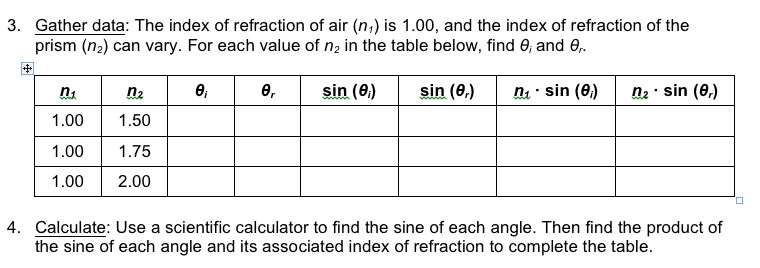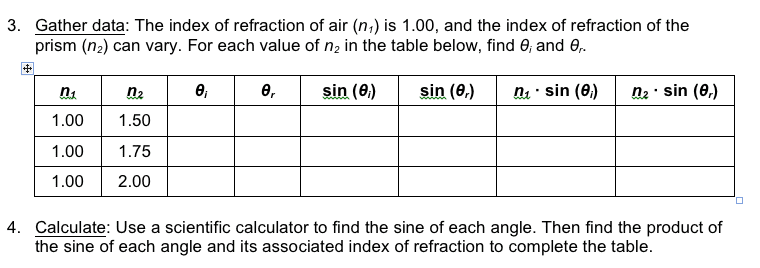# (Snell's Law) How do I find θi and θr?

Zoey

## Homework Statement

[/B]I am supposed to fill out a table with the values of θi and θr with the given information of n1 being 1.00 and n2 being 1.50 for the first row, and I am not quite sure how to do that just knowing the values that were given. I am also supposed to find the sine of θi and θr, and stuff like that but I think once I figure out how to find those values that will be easy. Just doing a basic search on the internet, it seems cross multiplication will need to come into play somewhere here, if so, what should I cross multiply?

## Homework Equations

[/B]Snell's Law: n2/n1 = Sin θi/Sin θr

## The Attempt at a Solution

[/B]I have tried various things such as just doing n2/n1, but I just don't seem to be getting relevant answers, because I don't think I know how to apply the equation properly. I would appreciate it if anyone has any suggestions! Thanks :)

*Note: I have a scientific calculator if that would be of helpLast edited:

drvrm
. Homework Statement I am supposed to fill out a table with the values of θi and θr with the given information of n1 being 1.00 and n2 being 1.50 for the first row, and I am not quite sure how to do that just knowing the values that were given. I am also supposed to find the sine of θi and θr, and stuff like that but I think once I figure out how to find those values that will be easy. Just doing a basic search on the internet, it seems cross multiplication will need to come into play somewhere here, if so, what should I cross multiply?

## Homework Equations

[/B]Snell's Law: n2/n1 = Sin θi/Sin θr

## The Attempt at a Solution

[/B]I have tried various things such as just doing n2/n1, but I just don't seem to be getting relevant answers, because I don't think I know how to apply the equation properly. I would appreciate it if anyone has any suggestions! Thanks :)

*Note: I have a scientific calculator if that would be of helpi think you can do it as in trigonometric function table sin of any angle is available - and a value given can invert the sin of the angle equivalent can also be found and tabulated.
you have n values known - so if choice of angle of incidence is made then the relation gives you sin of angle of refraction and from this value see the table of sin to get the angle of refraction.

Staff Emeritus
Homework Helper
Gold Member

## Homework Statement

[/B]I am supposed to fill out a table with the values of θi and θr with the given information of n1 being 1.00 and n2 being 1.50 for the first row, and I am not quite sure how to do that just knowing the values that were given. I am also supposed to find the sine of θi and θr, and stuff like that but I think once I figure out how to find those values that will be easy. Just doing a basic search on the internet, it seems cross multiplication will need to come into play somewhere here, if so, what should I cross multiply?

## Homework Equations

[/B]Snell's Law: n2/n1 = Sin θi/Sin θr

## The Attempt at a Solution

[/B]I have tried various things such as just doing n2/n1, but I just don't seem to be getting relevant answers, because I don't think I know how to apply the equation properly. I would appreciate it if anyone has any suggestions! Thanks :)

*Note: I have a scientific calculator if that would be of helpHello Zoey. Welcome to PF!

It would be helpful, if not absolutely necessary for you to give a bit of context to this thread and its problem. It's true that you did mention Snell's Law. Beyond that you need to say something regarding index of refraction and angles of incidence and refraction and as well, you need to define your variables .

You have not made it clear which quantities need to vary and which need to remain fixed.

(I will help with the thread title. - I have very limited powers.)

Zoey
Hello Zoey. Welcome to PF!

It would be helpful, if not absolutely necessary for you to give a bit of context to this thread and its problem. It's true that you did mention Snell's Law. Beyond that you need to say something regarding index of refraction and angles of incidence and refraction and as well, you need to define your variables .

You have not made it clear which quantities need to vary and which need to remain fixed.

(I will help with the thread title. - I have very limited powers.)

Here, if will be of help, is a picture of the problem itself. It is kind of fuzzy, but I think you should be able to read it. And thank you for trying to help!

#### Attachments

•reflect.png
12.3 KB · Views: 1,162
Staff Emeritus
Homework Helper
Gold Member
Here, if will be of help, is a picture of the problem itself. It is kind of fuzzy, but I think you should be able to read it. And thank you for trying to help!
The table you are to fill out:This looks suspiciously like some table that would be filled out during a lab exercise. Of course that would require access to prisms with those particular refractive indices. You would then be instructed to use some value for one of the angles (usually the incidence angle) and then measure the other angle. Plugging these values into this table should then help you verify Snell's Law.

This all suggests that there may be more information which you were given and which does not appear in this thread.

Zoey
The table you are to fill out:This looks suspiciously like some table that would be filled out during a lab exercise. Of course that would require access to prisms with those particular refractive indices. You would then be instructed to use some value for one of the angles (usually the incidence angle) and then measure the other angle. Plugging these values into this table should then help you verify Snell's Law.

This all suggests that there may be more information which you were given and which does not appear in this thread.

Yes, it makes sense that it would be filled out during a lab exercise as this is the table that I was supposed to fill out after completing a Gizmo (if you don't know what that is, it is like a virtual science lab).

Here is a picture of what they told me to fill in on the gizmo, other than that I have no other angles or anything like that.

Using the formula for Snell's Law, I attempted to go by that and do n2/n1, however, that only got me the answer for one of the values I need to fill in.

Here is a link to the actual "lab" I was supposed to complete, if that will help: https://www.explorelearning.com/index.cfm?method=cResource.dspView&ResourceID=608

Again, I really appreciate you trying to help and I apologize if I did not give you all the information you need to be able to help.#### Attachments

nasu
Well, then just go back to the Gizmo and measure the angles to fill the two columns. Then you do the calculations. The Gizmo includes a protractor for measuring the angles.
You cannot find the angles from Snell's law. The lab is supposed to prove Snell's law and not to use it.

•Zoey
Zoey
Well, then just go back to the Gizmo and measure the angles to fill the two columns. Then you do the calculations. The Gizmo includes a protractor for measuring the angles.
You cannot find the angles from Snell's law. The lab is supposed to prove Snell's law and not to use it.

So, are the angle of incidence and the angle of refraction what I would need to measure here? If so, I already have them measured. Is that what θi and θr stand for?

If that is the case, I think I figured out what I was missing and you have been a huge help! :)

Staff Emeritus
•